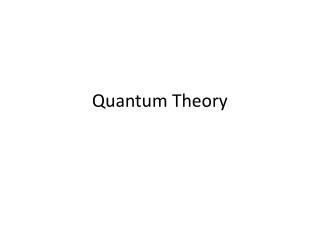DownloadDownload PresentationQuantum Theory

# Quantum Theory

Télécharger la présentation## Quantum Theory

- - - - - - - - - - - - - - - - - - - - - - - - - - - E N D - - - - - - - - - - - - - - - - - - - - - - - - - - -
##### Presentation Transcript

1. Quantum Theory

2. Planck’s Theory German physicist Max Karl Ernst Ludwig Planck • developed the concept of the “quantum” - the smallest unit of energy (The plural of “quantum” is “quanta”.) • The size of the energyquantumincreasesas the frequencyof the wave increasesand the size of the energy quantumdecreasesas the frequency of the wavedecreases. Therefore, energy is directlyproportional to frequency.

3. Mathematical representation of Planck’s Theory relating the energy of a quantum to the frequency of the wave E = h ν (energy) (Planck’s Constant) (frequency) 6.6262 x 10-34 J/sec

4. The Photoelectric Effect Albert Einstein Albert Einstein used Planck’s equation to explain another idea about light. In the photoelectric effect, electrons are ejected from the surface of a metal when light shines on the metal.

5. Strong red light is not capable of emitting electrons from the surface of sodium metal because red light has low frequency and therefore has low energy. A very weak beam of violet light is capable of emitting electrons from the same surface because violet light has high frequency and high energy.

6. Dual Nature of Radiant Energy: To explain the Photoelectric Effect, Albert Einstein proposed that: • acts like photons (A photon is a quantum of electromagnetic energy.) b. acts like light waves

7. Another Look at the Atom • Each element has its own fingerprint. Samples of all elements emit light when they are vaporized in an intense flame or when electricity is passed through their gaseous state. When this occurs, a spectrum is formed that contains only a few colors. A spectrum that contains only certain colors, or wavelengths, is called a line spectrum. EXAMPLES: Sodium  yellow light Nitrogen  orange light Mercury  blue light Hydrogen Neon red light Calcium Potassium  purple

8. Line Spectra

9. The Bohr Model of the Hydrogen Atom NielsBohr distinguished the relationship between wavelengths an element emits and its atomic structure. Through this connection, he explained the fact that each element is capable of emitting its own characteristic wavelengths of radiation. Bohr postulated that to get spectral lines, the energy of the electron in the atom must be quantized. This means that the electron allowed to have only certain orbits corresponding to different amounts of energy.

10. Bohr labeled each energy level, consequently each orbit, by a quantum number, n. For the lowest energy level where electrons have the most stability, or ground state, n = 1. This energy level corresponds to the orbit closest to the nucleus. When the electron absorbs the appropriate amount of energy, it jumps to a level of higher energy, called an excited level.